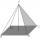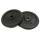Metal tube

Calculate the metal tube mass 8dm long with the outer radius 5cm and the inner radius 4.5cm and 1cm3 of this metal is 9.5g.

Result

m =  11.341 kg

Solution:Leave us a comment of example and its solution (i.e. if it is still somewhat unclear...):Be the first to comment!To solve this example are needed these knowledge from mathematics:

Do you know the volume and unit volume, and want to convert volume units? Do you want to convert mass units?

Next similar examples:

1. Metal pyramidFind the weight of a regular quadrilateral pyramid with a 5 cm length and 6,5 cm body height is made from material with density g/cm3.
2. Solid in waterThe solid weighs in air 11.8 g and in water 10 g. Calculate the density of the solid.
3. Common cylinderI've quite common example of a rotary cylinder. Known: S1 = 1 m2, r = 0.1 m Calculate : v =? V =? You can verify the results?
4. Cylinder - h2Cylinder volume is 2.6 liters. Base area is 1.3 dm2. Calculate the height of the cylinder.
5. LidWhat is the weight of concrete cover (lid) to round shape well with a diameter 1.8 m, if the thickness of the cover is 11 cm? 1 m3 of concrete weighs 2190 kg.
6. Iron castWhat is the weight of cast iron with volume 3575 cubic centimeters. The density of the cast iron is 7600 kg/m3
7. Density - simple exampleMaterial of density of 532 kg/m3 occupies a container volume of 79 cm3. What is its mass?
8. A pipeA radius of a cylindrical pipe is 2 ft. If the pipe is 17 ft long, what is its volume?
9. The potThe pot is a cylinder with a volume of V = 7l and an inner diameter of d = 20cm. Find its depth.
10. Railway wagonThe railway wagon holds 75 m3 load. Wagon can carry a maximum weight of 30 tonnes. What is the maximum density that may have material with which we could fill this whole wagon? b) what amount of peat (density 350 kg/m3) can carry 15 wagons?
11. ConvaHow many liters of water fit into the shape of a cylinder with a bottom diameter 20 cm and a height 45 cm?
12. Collect rain waterThe garden water tank has a cylindrical shape with a diameter of 80 cm and a height of 12 dm. How many liters of water will fit into the tank?
13. Tank and waterCylindrical tank were poured with 3.5 liters of water. If tank base diameter is 3 dm, how height is water level in?
14. Water levelHow high reaches the water in the cylindrical barell with a diameter of 12 cm if there is a liter of water? Express in cm with an accuracy of 1 decimal place.
15. Circular poolThe 3.6-meter pool has a depth of 90 cm. How many liters of water is in the pool?
16. The potDiameter of the pot 38 cm. The height is 30 cm. How many liters of water can fit in the pot?
17. Liters in cylinderDetermine the height at which level 24 liters of water in a cylindrical container having a bottom diameter 36 cm.